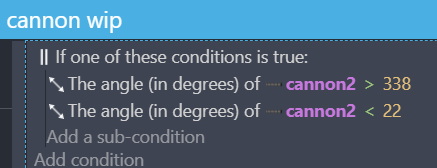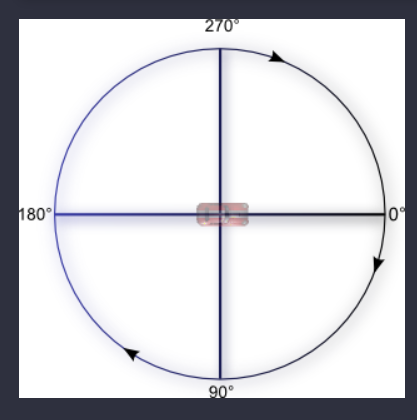# How do I limit firing area to specific zone

Big plane is ennemy small plane is player
I have canon (separate object) on back of wind shield of enemy plane
I want it to shoot player only when player is in range which you can see on the picture as red area
It would be ultra easy to do but problem is enemy plane can roate so it can fly straight up or straight down
And now how would i go about formula for checking that range?
I know right part of red area can be limit by distance of player from enemy plane
But i don’t know how to create that range if enemy plane can rotate

I’ve done something similar in the past, this is what I used:It helps when you have this example in mind:I didn’t realize that’s how it worked when I first started using GDevelop and it was very difficult trying to figure out angles lol.

You explained how to do something if angle of first object is in some angle range
Question is how do something if second object is in some angle range to first object considering first object can rotate

On provided picture big plane is flying to the left and red are detects to the right (which is behind big plane)
So if big plane would fly straight up that red area would need to detect towards bottom (which still would be behind big plane)

I think some formula is required here to use current big plane angle and do something using it with angle between big and smal planes

I found this to check angle between 2 objects

But i still have no idea how to create formula that would work with checking if small plane is behind big plane if big plane is rotating

EDIT PART BELOW
IDK if that would work but
Angle of big plane is equal to angle between big and smal plane
within some range

Like
BigPlane.Angle() = AngleBetween(BigPlane<>SmallPlane)+20
BigPlane.Angle() = AngleBetween(BigPlane<>SmallPlane)-20
And that gives range of 20 from 0 into each angle
At least i guess it should be more or less something like this

OK so
Front of Tree2 is from where it shoots bullets (for whatever reason it does not exactly to player position but to player direction but whatever)
PX and PY are position X and Y
Atan is angle between that two objects (imagine both objects are connect with a straight line and this is angle of that line)
Formula needs to be Player -Tree2 and not Tree2 - Player
If you go with Tree2 - Player you will get reverse + -

What is red is all negative numbers at that angle area
So everything below is positive +
If you go with Tree2 - Player instead of Player - Tree you will have - on bottom half while + on top half and it won’t work
To be clear Player would be small plane and Tree2 would be big plane

Now since (looking at 1st picture in this post) on left we have -180 then going 1° down would turn it into +180
And 1st higher angle from -180 would be -179 since less you lose it means bigger value
So going from -180 to +180 is not +1° but +360°
That’s why we need two possible angle ranges in If all of these conditions are true
Inside If one of these conditions is true
For Atan variable It needs to be ToDeg(atan2(GlobalVariable(PY), GlobalVariable(PX)))
NOT ToDeg(atan2(GlobalVariable(PX), GlobalVariable(PY))) or you will get bad result
Y variable needs to be first listed then X
And rest is either making range bigger or smaller depending on whatever is needed
In that event
Variables with + are if Tree2 is above player
Variables with - are if Tree2 is below player
Increasing 200 variable will increase area to the left (+ top - bottom)
Decreasing 160 variable will increase area to the right (+ top - bottom)
(considering + top is where Tree2 is facing so if it is facing top then increasing +200 to +220 will give you extra 20° for Tree2 to turn more left before it stop shooting)